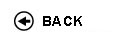Politecnico di Torino
01OUWOQ, 01OUWNG, 01OUWOT, 01OUWOV, 01OUWPE, 01OUWQW
Convex optimization and engineering applications
Master of science-level of the Bologna process in Electronic Engineering - Torino
Master of science-level of the Bologna process in Mathematical Engineering - Torino
Master of science-level of the Bologna process in Telecommunications Engineering - Torino
Espandi...
 Teacher Status SSD Les Ex Lab Tut Years teaching Calafiore Giuseppe CarloO2 ING-INF/04 40 20 0 0 10
 SSD CFU Activities Area context ING-INF/04 6 D - A scelta dello studente A scelta dello studente
Esclusioni:
01OVA; 01OVC; 01OVD; 01OVF; 01OVE; 01OUX; 01OUZ; 01OUY; 01OVB
 Subject fundamentals The course concentrates on recognizing and solving convex optimization problems that arise in engineering. Convex sets, functions, and optimization problems. Basics of convex analysis. Least-squares, linear and quadratic programs, semidefinite programming, minimax, extremal volume, and other problems. Optimality conditions, duality theory, theorems of alternative, and applications. Interior-point methods. Applications to signal processing, control, digital and analog circuit design, computational geometry, statistics, and mechanical engineering. Expected learning outcomes To give students the tools and training to recognize convex optimization problems that arise in engineering; to present the basic theory of such problems, concentrating on results that are useful in computation; to give students an understanding of how such problems are solved, and some experience in solving them. Prerequisites / Assumed knowledge Good knowledge of linear algebra, geometry, analysis and exposure to probability. Exposure to numerical computing, optimization, systems and control theory, and application fields is helpful but not required. Contents Introduction, convex sets and convex functions. Optimization problems in standard form, optimality criteria. Systems of linear equations, Least Squares (LS), Linear Programming (LP), Ell-one norm optimization, Chebychev approximation. Application examples: generation of force/torque via thrusters, uniform illumination of patch surfaces, etc. Quadratic Programming (QP) and Second Order Cone Programming (SOCP). Application examples: FIR filter design, antenna array design, sidelobe level minimization in beamforming. Linear Matrix Inequalities (LMI) and semidefinite programming (SDP). Geometric programming (GP). Introduction to software tools CVX and/or YALMIP. Applications: data-fitting, approximation and estimation, truss-structural design, transistor sizing, uncertain and robust Least Squares, Bounded-Real Lemma, passivity and applications in circuit theory. Geometrical problems: containment of poyhedra, classification, Lowner-John ellipsoids, linear discrimination, support vector machines. Interior-point methods. Focus seminars (mutually exclusive, to be offered alternatively over years): - LMIs in systems and control theory. - Sparse optimization and compressed sensing. - Convex optimization in Finance. - Convex optimization in algebraic geometry, global polynominal positivity (positivstellensaatz). - Network optimization, distributed optimization. Delivery modes Lab: The course requires about 30 hours teaching and 30 hours lab activity. Texts, readings, handouts and other learning resources 1. S. Boyd and L. Vandenberghe; Convex Optimization, Cambridge Univ. Press, 2004. 2. L. El Ghaoui, G. Calafiore; Optimization Models, Cambridge Univ. Press (in preparation) (draft to be made available to students) Assessment and grading criteria Written Examination) Programma definitivo per l'A.A.2015/16